### AME 301: Differential Equations, Vibrations and Control Homework 4

 B. GoodwineJ. Lucey Fall, 2003 Issued: September 10, 2003 Due: September 24, 2003 (US)25 September 2003 (UK)

Unless otherwise indicated, all problems are from the course text, Boyce and DiPrima, Elementary Differential Equations (and Boundary Value Problems), and each problem is worth 10 points.

1. (20 points - 2 points each) By inspection, determine for which of the following differential equations the solution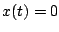is asymptotically stable. Unless otherwise indicated, you do not have to solve the equation.

1.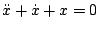.
2.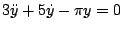.
3.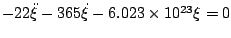.
4.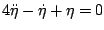.
5.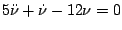.
6.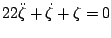.
7.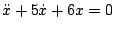(also find the general solution and verify your conclusion regarding stability).
8.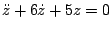.
9.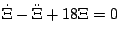(also find the general solution and verify your conclusion regarding stability).
10.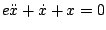.

2. (10 points) From the table presented in class on Monday, verify case 3 for distinct roots.

Note: It's impossible to have distinct roots with such a combination of signs'' is potentially an acceptable answer.

3. (10 points) From the table presented in class on Monday, verify case 4 for complex conjugate roots.

Note: It's impossible to have complex conjugate roots with such a combination of signs'' is potentially an acceptable answer.

4. (10 points) From the table presented in class on Monday, verify cases 5 for repeated roots.

Note: It's impossible to have repeated roots with such a combination of signs'' is potentially an acceptable answer.

5. (10 points) Use Rayleigh's method to determine the natural frequency of the system illustrated in the following figure.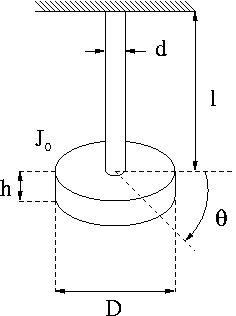6. Section 3.6 (10 points each)

1. 1
2. 2
3. 5 Hint: assume a constant additive term to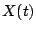.
4. 14

Last updated: September 10, 2003.
B. Goodwine (goodwine@controls.ame.nd.edu)
J. Lucey (jlucey@nd.edu)